# CAPACITY

##### HELLO, WELCOME BACK TO CLASS

Capacity is the amount of liquid (or other pourable substance) that an object can hold when it’s full. When a liquid, such as milk, is being described in gallons or quarts, this is a measure of capacity. Capacity is the amount of space a container, room etc. has to hold things or people. Capacity is the total amount of fluid that can be contained in a container. It is the word we use when we are measuring liquids. We might say: ‘What is the capacity of the liquid in that cup?’ to which an answer would be given in millilitres.

Volume: the amount of space that a substance or object contains or fills.

Litres is cubic centimeters (cm3)

An open cube of dimension 10 cm x 10 cm x 10 cm is a container of 1 litre capacity

1 litre (L) = 1,000 millilitres (ml)

1 L    = 1,000 ml                               ½ L    = 500 ml

¼ L    = 250 ml                                  ¾ L    = 750 ml

1/10 L = 100 ml                                  2 ¼ L = 2,250 ml

Examples on measurement of Capacity:

1. Express the following in ‘ml

(i) 19 l

(ii) 21 l 75 ml

(iii) 28 l 350 ml

Solution:

1 l = 1000 ml

(i)19 l

= 19 x 1000

= 19000 ml

(ii) 21 l 75 ml

= 21 x 1000 + 75

= 21000 + 75

= 21075 ml

(iii) 28 l 350 ml

= (28 x 1000) ml + 350 ml

= 28000 + 350

= 28350 ml

1. Convert the following into litres and millilitres.

(i) 75000 ml

(ii) 9485 ml

(iii) 23456 ml

Solution:

1000 ml = 1 l

(i) 75000 ml

= 75000 ml ÷ 1000

= 75 l

(ii) 9485 ml

= 9485 ml ÷ 1000

= 9 l 485 ml

(iii) 23456 ml

= 23456 ml ÷ 1000

= 23 l 456 ml

3. A water tank contains 2225 litres of water. During the day 975 l 325 ml of water is pumped out from the tank. How much water is left in the tank?

Solution:

Water in the tank = 2225 liters

Water pumped out = 975 liters 325 ml

So, water left =

Therefore water left = 1249 l 675 ml

1. A milkman sold 55 l 575 ml of milk on the first day, 40 l 480 ml on the second day and 60 l 825 ml on the third day. What quantity of milk did he sell during these three days?

Solution: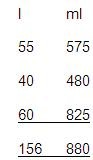Milk sold on first day          =

Milk sold on second day      =

Milk sold on third day          =                                    +

So, total milk sold              =

Therefore total milk sold      =                                               156 l 880 ml

1. A Dettol bottle contains 500 ml of Dettol. How much Dettol is contained in 12 dozen such bottles?

Solution:

Since one bottle contains Dettol = 500 ml

Therefore, 12 x 12 bottles contain Dettol = 500 ml x 12 x 12

= 500 ml x 144

= 72000 ml

= 72 litres

1. A drum contains 12 litres 156 ml of varnish. It is supplied to 12 shops equally. How much varnish does each shop get?

Solution:

Varnish distributed to 12 shops = 12 l 156 ml

Varnish given to 1 shop = (12 l 156 ml) ÷ 12

= 12156 ml ÷ 12

= 1013 ml

= 1 l 13 ml

Each shop will get varnish = 1 l 13 ml

Multiplication and Division of Litres:

Examples: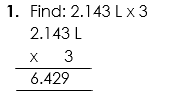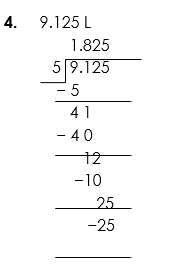WORD PROBLEMS ON CAPACITY

Examples:

1. How many 4 litre buckets are needed to fill a tank holding 108 litres?

Solution: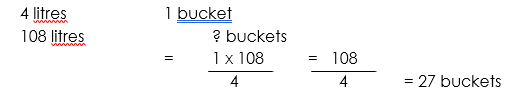1. A car travels 8 km using 1 litre of petrol. How much petrol is needed to travel 152 km?

Solution: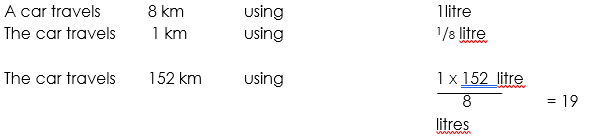1. How many half-litres are there in:                                                                                                                        a. 1 ½ L     b. 3 ½ L                    c.   5 ½ L                    d.  12 ½ L                  e.  10 L

Solution: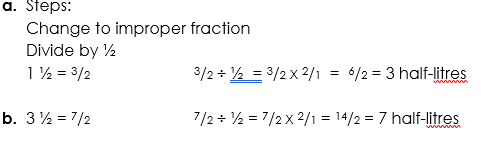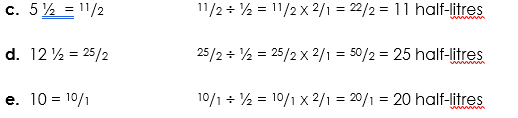1. What is the capacity of 48 drums if each drum contains 357 litres of palm oil.

Solution:

1 drum contains                 357 litres                    of palm oil

48 drums contain               (357 x 48) litres =         17,136 litres           of palm oil.

1000 cm3 = 1 litre = 1000 ml

1 cm3 = 1 ml

29 cl = 290 ml       35 cl = 350 ml.

Quiz

1. Express the following in ‘ml

(i) 19 l

(ii) 21 l 75 ml

(iii) 28 l 350 ml

1. A drum contains 12 litres 156 ml of varnish. It is supplied to 12 shops equally. How much varnish does each shop get?
1. A milkman sold 55 l 575 ml of milk on the first day, 40 l 480 ml on the second day and 60 l 825 ml on the third day. What quantity of milk did he sell during these three days?
How Can We Make ClassNotesNG Better? - CLICK to Tell Us Now!